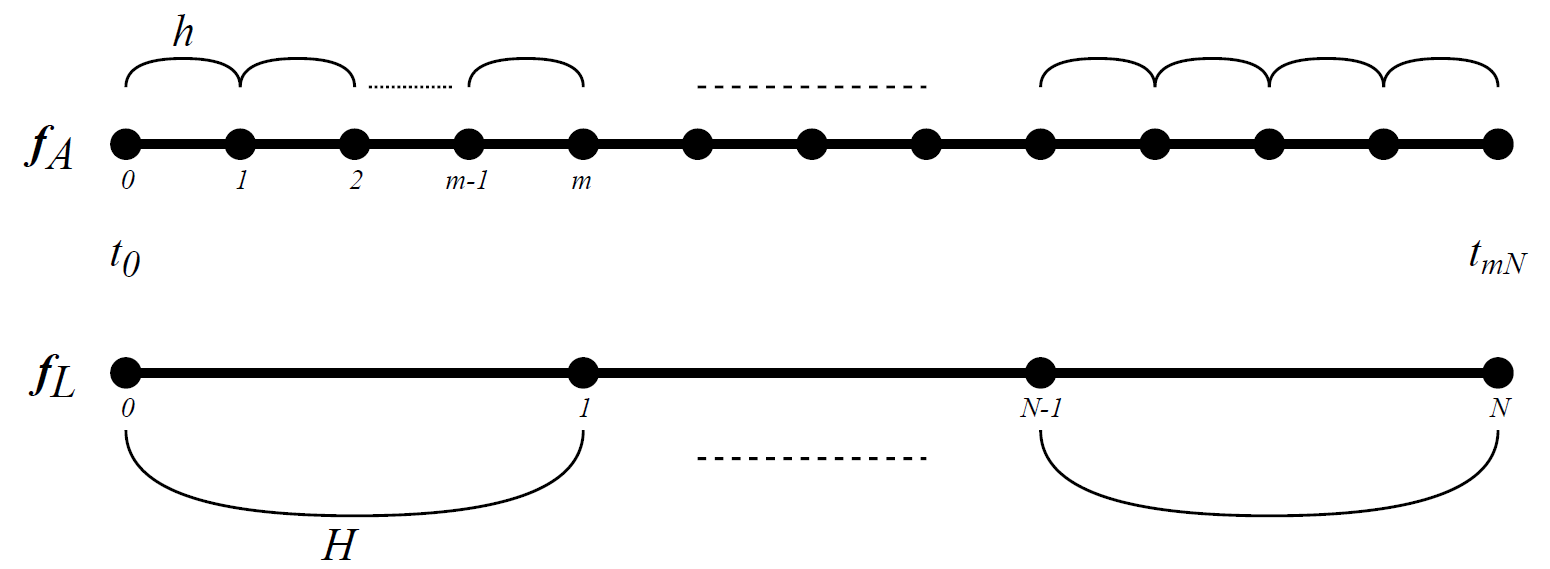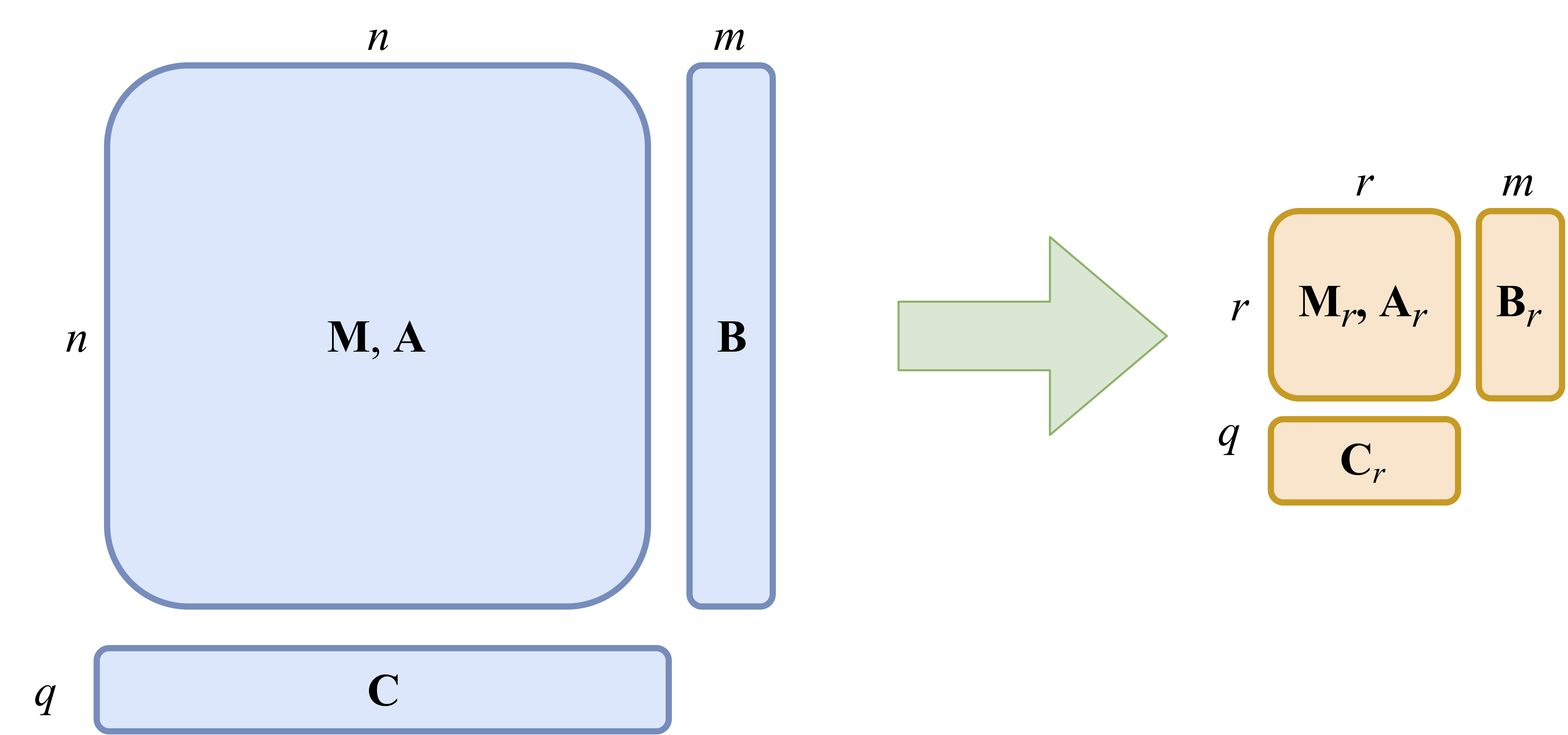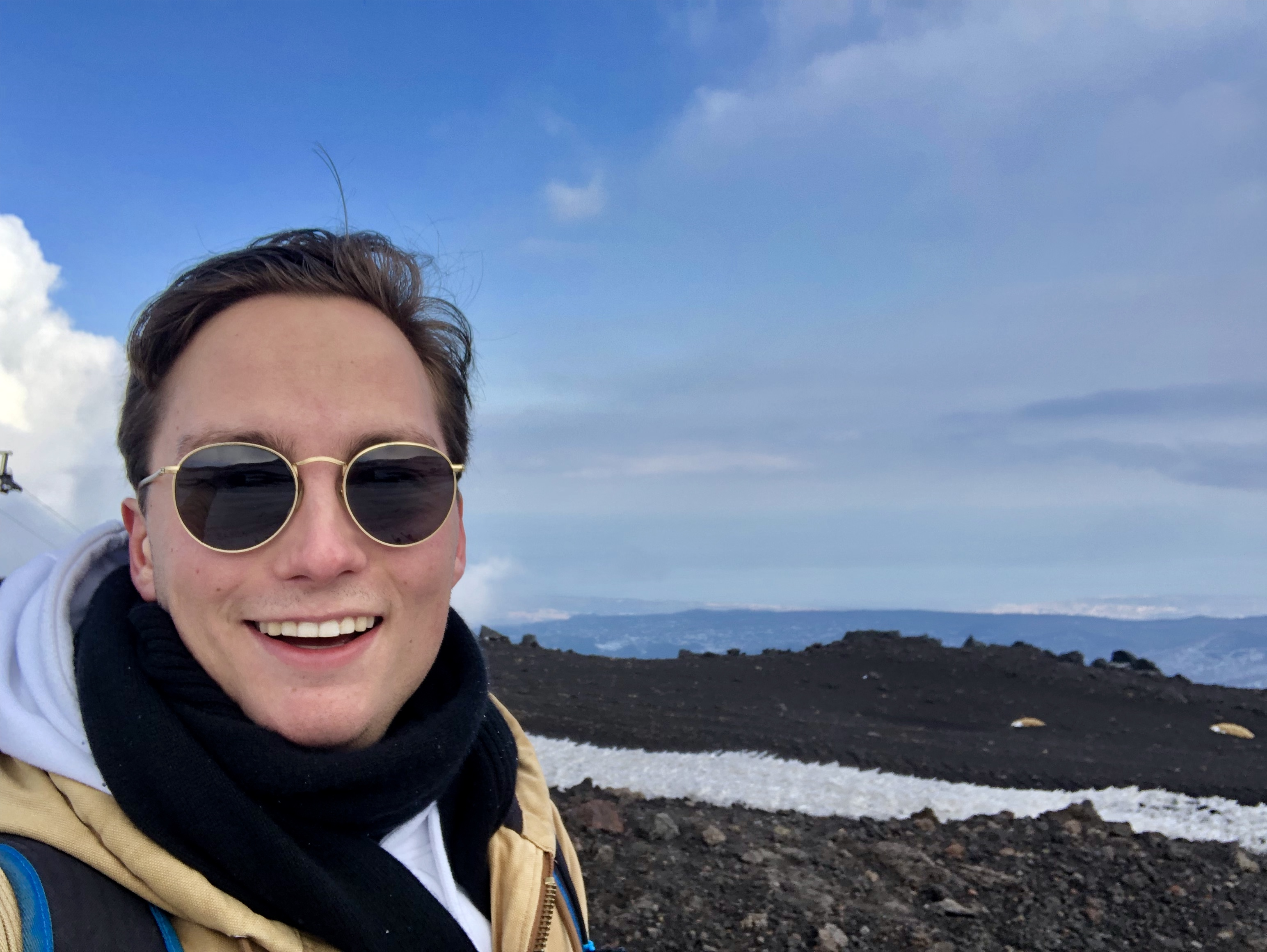# Under the Volcano – Heat in Microchips

As ESR-5 in the ROMSOC project I work at the Bergische Universität Wuppertal (Germany) in the picturesque surroundings of das Bergisches Land and at ST Microelectronics at the foot of Mount Etna in Catania (Italy). After having spent my first three months in Wuppertal, I am now living in Catania for six months. In a stark contrast to the colossal size of the ever looming mount Etna, my research takes me into the miniature world of nanoelectronics.

Microchip details are in the order of meters, one millionth of a millimetre. On that scale a human hair looks like a giant tree. Now many phenomena (electro mechanical, thermal, quantum physical) occur inside microchips. In this blog I will focus on thermal aspects. Given the nano-scale of the details it is impossible to perform any measurements inside a working microchip. To understand and try to improve processes inside microchips we convert them into mathematical equations and apply a combination of multirate (MR) and model order reduction (MOR) techniques. Both techniques are well known individually but combining them is novel.

Our goal is to build a simulation of what happens inside a working microchip to help microchip designers create better products.

### The Problem: Simulating A Large Coupled System

In the simulation of nanoelectronics there are a great many things to consider. Whilst trying to accurately model the natural phenomena happening inside a microchip one should also safeguard the feasibility of this models numerical solutions. The focus of this project lies on the simulation of large coupled systems with di erent intrinsic time scales.

So let us start at the beginning and look at the origins of these large coupled systems. A microchip is essentially a circuit of many di fferent components, e.g. resistors or transistors, that interact with each other. These interactions are modelled by means of equations and together they form a system of equations. The equations governing the heat of components and the circuit are coupled together, resulting in a coupled system of equations:

Where is the state vector of the coupled system,  the input and the output vector. Furthermore, we consider the system, the input and the output to be linear for now.
As these circuits get more and more intricate these systems continue to grow in size. For modern day microchips these systems can consist of millions of equations. As one could imagine this would become quite unfeasible for straightforward simulation.

### MR and MOR

The objective of this project is to combine MR and MOR to drastically improve the simulation speed. MR can exploit the di erent dynamical time scales of subsystems to improve the overall computational efficiency, without losing the global solution accuracy. MOR aims to reduce the size of subsystems and decrease the numerical eff ort for each time step whilst maintaining a certain level of accuracy.

### MR

Consider the previously mentioned system of circuit equations and heat equations, combined in one generalised system.

with . Since two phenomena act on separate time scales, (circuit equations evolve very fast compared to the slow heat equations) the system can be naturally split into two systems.

Where denote the fast changing, active components and
the slow changing, latent components of . By using a multirate method this system can be integrated using a macro time step for the latent subsystem and a micro time step for the active subsystem, as illustrated in the figure below.So MR divides the system into subsystems with di fferent dynamic scales. This results in subsystems with similar intrinsic characteristics, which makes them suitable for model order reduction.

### MOR

In MOR the goal is to try to approximate the output  of a large system  of  equations. This approximation is done by creating a smaller system of  equations. This smaller system   should be constructed in such a way that the di fference between its output,  and the original, , is small, while is also much smaller than .

In the context of circuit simulation this smaller system is used to obtain faster simulations with results that approximate the full simulation to a certain degree of accuracy. A general form of MOR can be described by choosing suitable matrices and , with  and biorthonormal, to create the following projection:

Which can be expressed in mathematical form by### The Solution: Combining MR and MOR

To give an intuitive example of how to combine these two techniques consider a multirate system as de fined by

Note that  is considered to be a linear. Now we can apply model order reduction to    by constructing matrices and . This results in the system

For now this simple example serves as an apt illustration. However, as the dimension of the output variable  is still equal to the dimension of the coupling interface we are not quite there yet, but those technicalities will be a story for another blog post.

### The Research Project

The main objective of this project is to combine MR and MOR in an industrial setting. This means that instead of the simple linear system given as an example, more complex systems will be considered, e.g. non-linear time-dependent systems or combinations of a variety of diff erent subsystems linked together for which diff erent types of MR and MOR techniques need to be considered.

Besides the combination of the two techniques other goals include: defi ning error estimates linked to the accuracy requirements of the iteration level of the optimisation flow, the preservation of overall properties of the system and stability of the dynamic iteration process.

I hope that this brief introduction to my research topic has given you some insight into my daily activities. To follow the progress of my fellow researchers and me please keep a sharp eye on the ROMSOC website and follow us on Twitter and Facebook!
For now tschüss and arrivederci.
Marcus Bannenberg